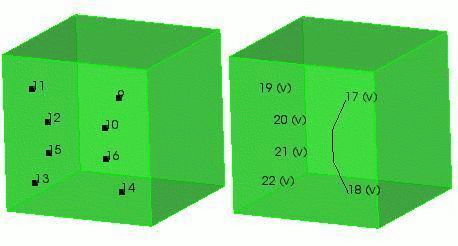# Partitioned Surfaces

There are several forms of the command to partition a surface. A surface may be partitioned using hard points, curves, polylines, mesh edges, mesh faces or mesh triangles.

## Partitioning with Vertices and Nodes

### Partitioning with Hard Points

There are two methods of partitioning a surface using vertices and nodes. The first method is to create a set of hard points using nodes, vertices, or coordinates that constrain the mesh to particular points on the surface. The syntax is:

Partition Create Surface <id> Vertex <id_list> [Individual]

Partition Create Surface <id> Node <id_list> [Individual]

### Partitioning with Polylines

The second method is to define a polyline using a set of vertices or coordinates. This method splits the surface using a polyline defined by the a list of positions specified as either coordinate triples, or existing vertices. The polyline is projected to the surface to define the curve for splitting the surface. If only one position is specified a zero-length curve with a single vertex will be created The syntax is identical to above WITHOUT the individual option.

Partition Create Surface <id> Vertex <id_list>

Partition Create Surface <id> Position <x> <y> <z> [[Position] <x> <y> <z> ...]

In the following simple example, the surface is partitioned using both methods. On the left half of the object, the surface is partitioned using the individual option (vertices 11 12 15 13). On the right half, a polyline is used (vertices 9 10 16 14). All of the free vertices can then be deleted, leaving the virtual curves shown in the second picture. Vertices 19 20 21 and 22 are all zero-length curves. The small 'v' in parentheses is to indicate that it is virtual geometry. The resulting mesh is shown in the third picture. Notice that the polyline constrains the entire curve to the mesh, while the hardpoints constrain only that individual point.Figure 1. Partitioning a Surface Using Vertices

## Partitioning with Curves

This form of the command splits the existing surface into several surfaces by creating curves that approximate the projection of the specified existing curves onto the surface. The syntax is:

Partition Create Surface <id> Curve <id_list>

## Partitioning with Mesh Edges

Meshed surfaces may be partitioned with mesh edges. The specified mesh edges must be owned by the surface to be partitioned. The shape of the curve(s) used to split the surface is specified by a set of mesh edges.

If the split location is specified by a series of mesh edges, and the specified mesh edges form a closed loop, the node option may be used to control which node the vertex is created at.

Partition Create Surface <id> Edge <id_list> [Node <node_id>]

## Partitioning with Faces or Triangles

Surfaces may also be partitioned by specifying a list of triangles or faces (quads). The boundary of the list will automatically be detected and new curves and vertices created at the appropriate locations. Curves are created from the mesh edges and used to split the surface. The surface mesh is split and assigned to the appropriate surface partitions.

Partition Create Surface <id> Face|Tri <id_list>